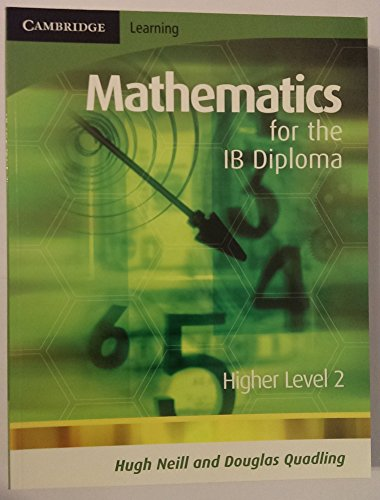# Mathematics for the IB Diploma Higher Level 2 by Douglas Quadling

In Stock
\$20.99
+10% GST
Mathematics for the IB Diploma has been extensively reviewed by IB Teachers to create reliable resources for studying IB Mathematics
Only 1 left

## Mathematics for the IB Diploma Higher Level 2 Summary

### Mathematics for the IB Diploma Higher Level 2 by Douglas Quadling

Specifically written to cover the new IB Mathematics syllabuses, these books provide a weath of practice material and have been extensively tested in classrooms. They include: full coverage of the IB syllabus; past examination questions; revision sections at regular intervals; and a full answer key. The books also describe graphical calculator methods as required by the IB syllabus.

### Why buy from World of BooksOur excellent value books literally don't cost the earthFree delivery in AustraliaEvery used book bought is one saved from landfill

Introduction; 1. Implication and induction; 2. Composite and inverse functions; 3. Transforming graphs; 4. The modulus function; Review exercise 1; 5. Relations between trigonometric functions; 6. Trigonometric equations; 7. Circular functions; 8. Reciprocal functions; 9. Inverse circular functions; Review exercise 2; 10. Continuous random variables; 11. The normal distribution;Review exercise 3; 12. Extending differentiation and integration; 13. Differentiating exponentials and logarithms; 14. Differentiating products and quotients; 15. Volumes of revolution; Review exercise 4; 16. Vectors; 17. Scalar products of vectors; 18. Sets of linear equations; 19. Lines in 3-dimensions; Review exercise 5; 20. The chain rule; 21. Differentiating circular functions; 22. Integrating products; 23. Differential equations; Review exercise 6; 24. Planes; 25. The vector product;Review exercise 7; 26. Integration by substitution; 27. Curves defined implicitly; 28. Differentiating inverse trigonometric functions; Review exercise 8; 29. Matrices; 30. Inverse matrices; 31. Determinants; Review exercise 9; 32. Complex numbers; 33. The complex plane; 34. Complex numbers in polar form; 35. De Moivre's theorem; 36. Further trigonometry; Review exercise 10; Answers; Index.

GOR002677721
Mathematics for the IB Diploma Higher Level 2 by Douglas Quadling